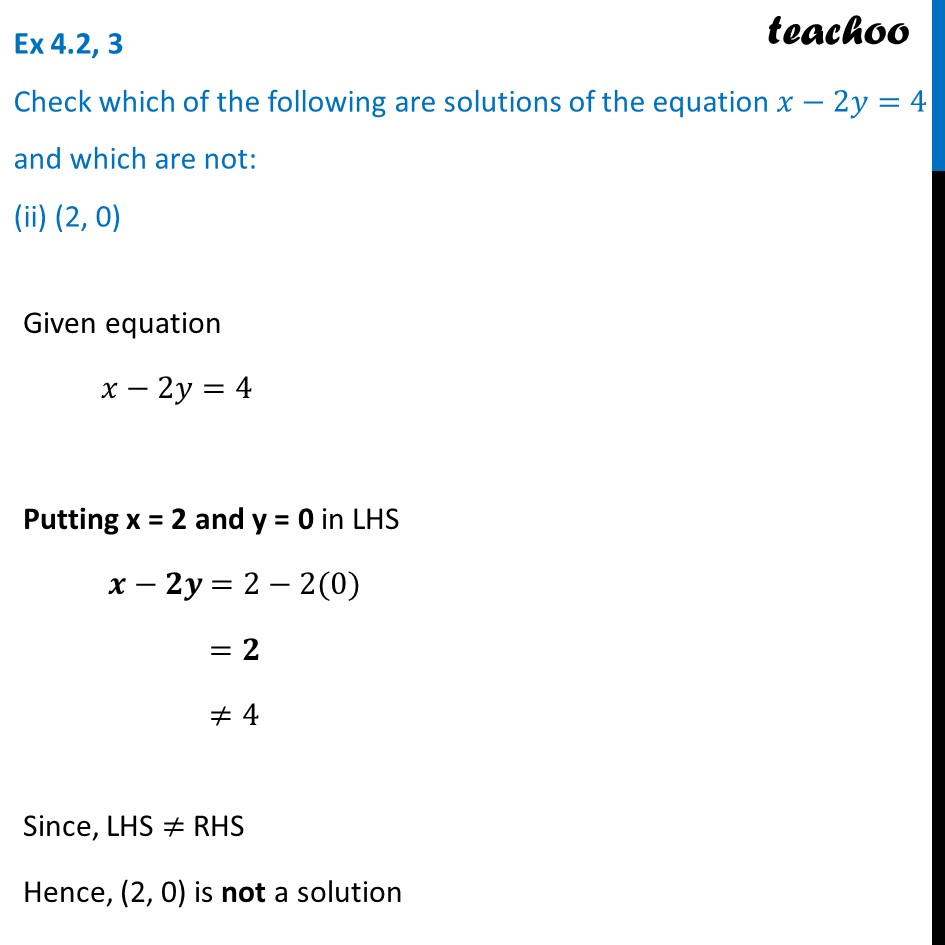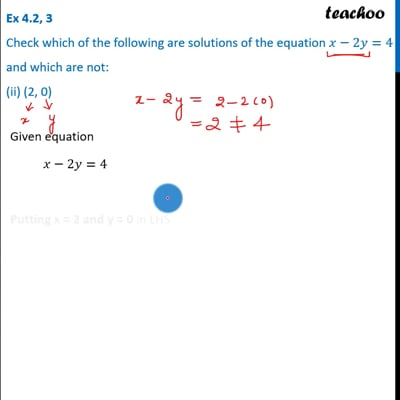Ex 4.2

Chapter 4 Class 9 Linear Equations in Two Variables
Serial order wiseThis video is only available for Teachoo black users

Introducing your new favourite teacher - Teachoo Black, at only ₹83 per month

### Transcript

Ex 4.2, 3 Check which of the following are solutions of the equation 𝑥−2𝑦=4 and which are not: (ii) (2, 0) Given equation 𝑥−2𝑦=4 Putting x = 2 and y = 0 in LHS 𝒙−𝟐𝒚 =2−2(0) =𝟐 ≠4 Since, LHS ≠ RHS Hence, (2, 0) is not a solution